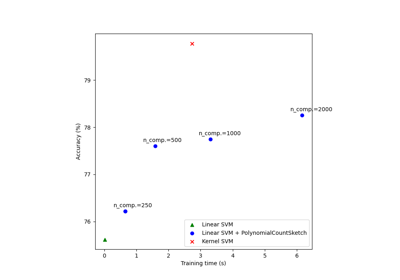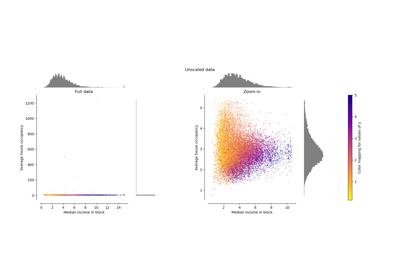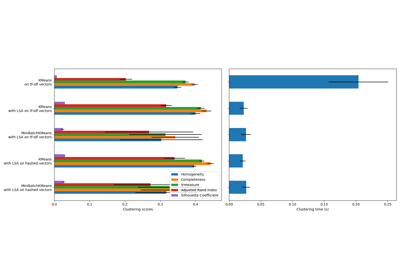# sklearn.preprocessing.Normalizer¶

class sklearn.preprocessing.Normalizer(norm='l2', *, copy=True)[source]

Normalize samples individually to unit norm.

Each sample (i.e. each row of the data matrix) with at least one non zero component is rescaled independently of other samples so that its norm (l1, l2 or inf) equals one.

This transformer is able to work both with dense numpy arrays and scipy.sparse matrix (use CSR format if you want to avoid the burden of a copy / conversion).

Scaling inputs to unit norms is a common operation for text classification or clustering for instance. For instance the dot product of two l2-normalized TF-IDF vectors is the cosine similarity of the vectors and is the base similarity metric for the Vector Space Model commonly used by the Information Retrieval community.

Read more in the User Guide.

Parameters:
norm{‘l1’, ‘l2’, ‘max’}, default=’l2’

The norm to use to normalize each non zero sample. If norm=’max’ is used, values will be rescaled by the maximum of the absolute values.

copybool, default=True

Set to False to perform inplace row normalization and avoid a copy (if the input is already a numpy array or a scipy.sparse CSR matrix).

Attributes:
n_features_in_int

Number of features seen during fit.

New in version 0.24.

feature_names_in_ndarray of shape (n_features_in_,)

Names of features seen during fit. Defined only when X has feature names that are all strings.

New in version 1.0.

normalize

Equivalent function without the estimator API.

Notes

This estimator is stateless and does not need to be fitted. However, we recommend to call fit_transform instead of transform, as parameter validation is only performed in fit.

For a comparison of the different scalers, transformers, and normalizers, see examples/preprocessing/plot_all_scaling.py.

Examples

>>> from sklearn.preprocessing import Normalizer
>>> X = [[4, 1, 2, 2],
...      [1, 3, 9, 3],
...      [5, 7, 5, 1]]
>>> transformer = Normalizer().fit(X)  # fit does nothing.
>>> transformer
Normalizer()
>>> transformer.transform(X)
array([[0.8, 0.2, 0.4, 0.4],
[0.1, 0.3, 0.9, 0.3],
[0.5, 0.7, 0.5, 0.1]])


Methods

 fit(X[, y]) Only validates estimator's parameters. fit_transform(X[, y]) Fit to data, then transform it. get_feature_names_out([input_features]) Get output feature names for transformation. get_params([deep]) Get parameters for this estimator. set_output(*[, transform]) Set output container. set_params(**params) Set the parameters of this estimator. transform(X[, copy]) Scale each non zero row of X to unit norm.
fit(X, y=None)[source]

Only validates estimator’s parameters.

This method allows to: (i) validate the estimator’s parameters and (ii) be consistent with the scikit-learn transformer API.

Parameters:
X{array-like, sparse matrix} of shape (n_samples, n_features)

The data to estimate the normalization parameters.

yIgnored

Not used, present here for API consistency by convention.

Returns:
selfobject

Fitted transformer.

fit_transform(X, y=None, **fit_params)[source]

Fit to data, then transform it.

Fits transformer to X and y with optional parameters fit_params and returns a transformed version of X.

Parameters:
Xarray-like of shape (n_samples, n_features)

Input samples.

yarray-like of shape (n_samples,) or (n_samples, n_outputs), default=None

Target values (None for unsupervised transformations).

**fit_paramsdict

Returns:
X_newndarray array of shape (n_samples, n_features_new)

Transformed array.

get_feature_names_out(input_features=None)[source]

Get output feature names for transformation.

Parameters:
input_featuresarray-like of str or None, default=None

Input features.

• If input_features is None, then feature_names_in_ is used as feature names in. If feature_names_in_ is not defined, then the following input feature names are generated: ["x0", "x1", ..., "x(n_features_in_ - 1)"].

• If input_features is an array-like, then input_features must match feature_names_in_ if feature_names_in_ is defined.

Returns:
feature_names_outndarray of str objects

Same as input features.

get_params(deep=True)[source]

Get parameters for this estimator.

Parameters:
deepbool, default=True

If True, will return the parameters for this estimator and contained subobjects that are estimators.

Returns:
paramsdict

Parameter names mapped to their values.

set_output(*, transform=None)[source]

Set output container.

See Introducing the set_output API for an example on how to use the API.

Parameters:
transform{“default”, “pandas”}, default=None

Configure output of transform and fit_transform.

• "default": Default output format of a transformer

• "pandas": DataFrame output

• None: Transform configuration is unchanged

Returns:
selfestimator instance

Estimator instance.

set_params(**params)[source]

Set the parameters of this estimator.

The method works on simple estimators as well as on nested objects (such as Pipeline). The latter have parameters of the form <component>__<parameter> so that it’s possible to update each component of a nested object.

Parameters:
**paramsdict

Estimator parameters.

Returns:
selfestimator instance

Estimator instance.

transform(X, copy=None)[source]

Scale each non zero row of X to unit norm.

Parameters:
X{array-like, sparse matrix} of shape (n_samples, n_features)

The data to normalize, row by row. scipy.sparse matrices should be in CSR format to avoid an un-necessary copy.

copybool, default=None

Copy the input X or not.

Returns:
X_tr{ndarray, sparse matrix} of shape (n_samples, n_features)

Transformed array.

## Examples using sklearn.preprocessing.Normalizer¶Scalable learning with polynomial kernel approximation

Scalable learning with polynomial kernel approximationCompare the effect of different scalers on data with outliers

Compare the effect of different scalers on data with outliersClustering text documents using k-means

Clustering text documents using k-means